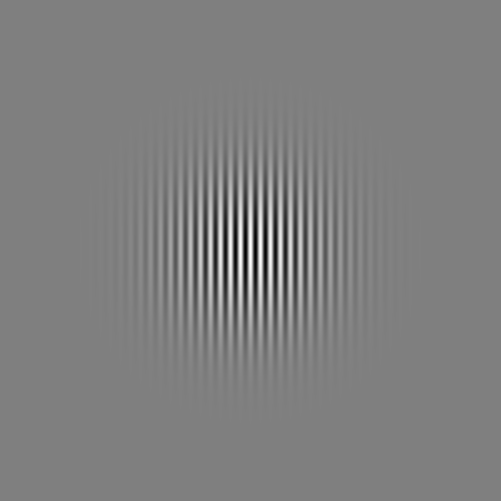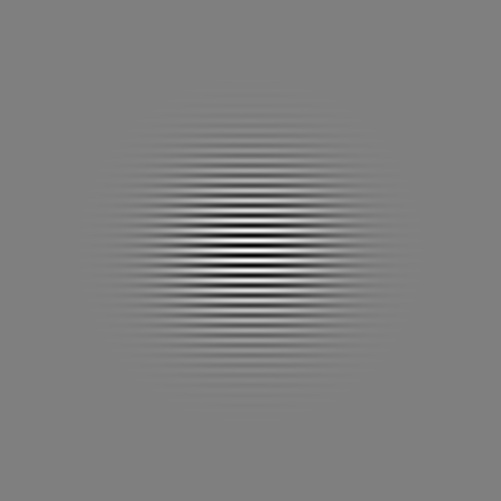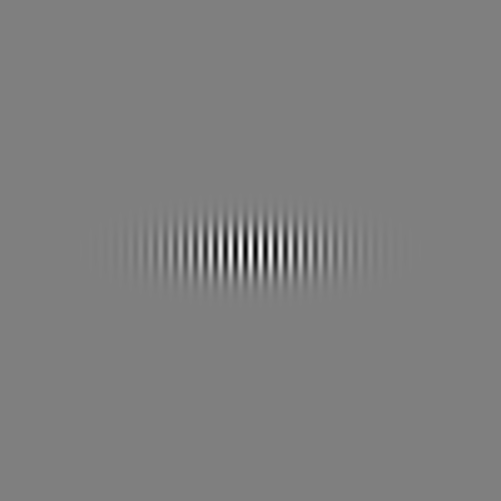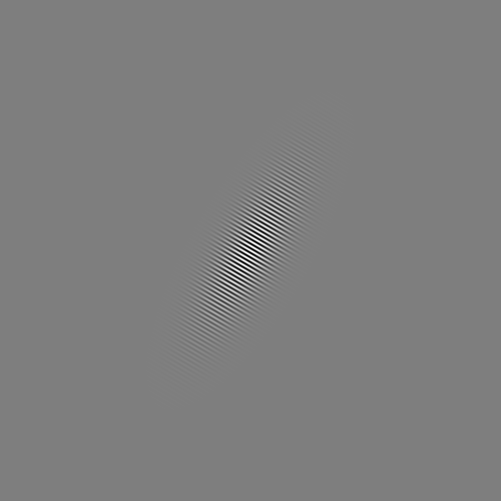# aurellem ☉

Gabor filters were invented by the same guy who invented holograms.

They work well as edge detectors and are related to the human visual system.

```(ns cortex.gabor
(:import org.opencv.core.CvType)
(:import java.awt.image.BufferedImage)
(:import ij.ImagePlus)
(:import org.opencv.core.Mat)
(:use (cortex world sense util vision import))
(:import com.jme3.post.SceneProcessor)
(:import (com.jme3.util BufferUtils Screenshots))
(:import java.nio.ByteBuffer)
(:import java.awt.image.BufferedImage)
(:import (com.jme3.renderer ViewPort Camera))
(:import (com.jme3.math ColorRGBA Vector3f Matrix3f Vector2f))
(:import com.jme3.renderer.Renderer)
(:import com.jme3.app.Application)
(:import com.jme3.texture.FrameBuffer)
(:import (com.jme3.scene Node Spatial)))

(cortex.import/mega-import-jme3)
(use  'clojure.math.numeric-tower)
"Load the opencv native library. Must be called before any OpenCV
stuff is used."
[]

(defn gabor-kernel
([sigma wavelength theta]
(gabor-kernel sigma wavelength theta 1 0))
([sigma wavelength]
(gabor-kernel sigma wavelength 0 1 0))
([sigma wavelength theta aspect-ratio phase-offset]

;; first, find the size of the kernel which is required
(let [square #(expt % 2)
rotated (fn [[x y]]
[(+ (* x (Math/cos theta)) (* y (Math/sin theta)))
(- (* y (Math/cos theta))  (* x (Math/sin theta)))])

gaussian (fn [[x y]]
(let [[x' y'] (rotated [x y])]
(Math/exp (- (/ (+ (square x')
(square (* aspect-ratio y')))
(* 2 (square sigma)))))))
sinusoid (fn [[x y]]
(let [[x' y'] (rotated [x y])]
(Math/cos
(+ (* 2 Math/PI (/ x' wavelength))
phase-offset))))

half-width
(let [std-dev-capture 5]
(max
(int (* std-dev-capture (/ sigma aspect-ratio)))
(int (* std-dev-capture sigma))
(int (* std-dev-capture (/ aspect-ratio sigma)))))

grid (let [axis (range (- half-width) (inc half-width))]
(for [y (reverse axis) x axis] (vector x y)))

scale (reduce + (map gaussian grid))

gabor (fn [[x y :as coord]]
(* (sinusoid coord) (gaussian coord) (/ scale)))

mat-width (+ 1 (* 2 half-width))
mat (Mat. mat-width mat-width CvType/CV_32F)]

(.put mat 0 0 (float-array (map gabor grid)))
mat)))

(defn draw-kernel! [kernel img-path]
(let [output img-path
size (.size kernel)
width (int (.width size))
height (int (.height size))
tmp-array (float-array (* width height))]

(.get kernel 0 0 tmp-array)

;; find overall dynamic range of the filter
(let [vals (vec tmp-array)
low (apply min vals)
high (apply max vals)
scaled-vals (map #(* 255 (- % low) (/ (- high low))) vals)
new-mat (Mat. height width CvType/CV_32F)]
(.put new-mat 0 0 (float-array scaled-vals))
(org.opencv.highgui.Highgui/imwrite output new-mat))))

;; some cool examples
``````(def img-base "/home/r/proj/cortex/images/")

(draw-kernel! (gabor-kernel 50 10 0 1 0)
(str img-base "gabor-50-10.png"))
``````(draw-kernel! (gabor-kernel 50 10 (/ Math/PI 4) 1 0)
(str img-base "gabor-50-10-pi-over-4.png"))
``````(draw-kernel! (gabor-kernel 50 10 (/ Math/PI 2) 1 0)
(str img-base "gabor-50-10-pi-over-2.png"))
``````(draw-kernel! (gabor-kernel 50 50 0 1 0)
(str img-base "gabor-50-50.png"))
``````(draw-kernel! (gabor-kernel 50 10 0 3 0)
(str img-base "gabor-50-10-0-3.png"))
``````(draw-kernel! (gabor-kernel 50 4 (/ Math/PI 3) 3 0)
(str img-base "gabor-50-4-pi-over3-3.png"))
```
```(defn show-kernel [kernel]
(let [img-path "/home/r/proj/cortex/tmp/kernel.png"]
(draw-kernel! kernel img-path)
(view (ImagePlus. img-path))))

(defn print-kernel [kernel]
(println (.dump kernel)))

(import com.aurellem.capture.Capture)

(import java.io.File)

(def base "/home/r/proj/cortex/render/gabor-1/")

(defn brick-wall-game-run [record?]
(let [capture-dir (File. base "main")]

(.mkdir (File. base "main"))
(doto
(world
(doto (Node.) (.attachChild (floor*))
(.attachChild (brick-wall*))
)
{"key-f" (fn [game value]
(if (not value) (add-element game (brick-wall*))))
"key-space" (fire-cannon-ball )}
(fn [world]
(position-camera
world
(Vector3f. 1.382548, 4.0383573, 5.994235)
(Quaternion. 0.0013082094, 0.98581666,
-0.1676442, 0.0076932586))

;;(speed-up world)

(if record?
(Capture/captureVideo
world capture-dir))

(fn [& _]))
(.start))))

(defn convolve-preview [kernel]
(let [input "/home/r/proj/cortex/render/gabor-1/main/0000032.png"

output "/home/r/ppp.png"

;;kernel (gabor-kernel 10 1 (/ Math/PI 2) 10 0)

new-mat (Mat.)

]

(org.opencv.imgproc.Imgproc/filter2D
i new-mat CvType/CV_32F kernel)

(org.opencv.highgui.Highgui/imwrite "/home/r/ppp.png" new-mat)

(view (ImagePlus. input))
(view (ImagePlus. output))))

(use 'clojure.java.shell)

(defn apply-gabor [kernel source dest]
new-mat (Mat.)]

(println dest)
(if (not (.exists (File. dest)))
(do
(org.opencv.imgproc.Imgproc/filter2D
i new-mat CvType/CV_32F kernel)
(org.opencv.highgui.Highgui/imwrite dest new-mat)
(println "mogrify" "-modulate" "1000%" dest)
(sh "mogrify" "-modulate" "1000%" dest)))))

(import java.io.File)

(defn images [path]
(sort (rest (file-seq (File. path)))))

(defn pics [file]
(images (str base file)))

(defn generate-gabor-images [kernel name]
(draw-kernel! kernel (str base name ".png"))

(.mkdir (File. (str base name)))

(let [main (map #(.getCanonicalPath %) (pics "main"))
targets (map #(str base name "/" (format "%07d.png" %))
(range 0 (count main)))]
(dorun (pmap (partial apply-gabor kernel) main targets))))

(def banks
[[(gabor-kernel 2.8 3.5) "bank-1-1"]
[(gabor-kernel 2.8 3.5 (/ Math/PI 2)) "bank-1-1-rot"]

;;   [(gabor-kernel 3.6 4.6) "bank-1-2"]
;;   [(gabor-kernel 4.5 5.6) "bank-2-1"]
;;   [(gabor-kernel 6.3 7.9) "bank-3-1"]
;;   [(gabor-kernel 7.3 9.1) "bank-3-2"]

[(gabor-kernel 12.3 15.4) "bank-6-1"]

;;   [(gabor-kernel 17 21.2) "bank-8-1"]
;;   [(gabor-kernel 18.2 22.8) "bank-8-2"]
])

(defn make-all-images []
(dorun (map (partial apply generate-gabor-images) banks)))

(defn compile-left-right []
(.mkdir (File. (str base "left-right")))
(let [main (pics "main")
left (pics "bank-1-1")
right (pics "bank-1-1-rot")
left-kernel (repeat 20000 (File. (str base "bank-1-1.png")))
right-kernel (repeat 20000 (File. (str base "bank-1-1-rot.png")))
targets (map
#(File. (str base "left-right/" (format "%07d.png" %)))
(range 0 (count main)))]

(dorun
(pmap
(comp
(fn [[main left right left-kernel right-kernel target]]
(println target)
(if (not (.exists (File. target)))
(sh "convert"
"-size" "1940x515" "xc:white"
main      "-geometry" "+0+0"    "-composite"
left "-geometry" "+650+0"   "-composite"
right     "-geometry" "+1300+0"  "-composite"
left-kernel      "-geometry" "+960+485"  "-composite"
right-kernel      "-geometry" "+1610+485"  "-composite"
target)))
(fn [& args] (map #(.getCanonicalPath %) args)))
main left right left-kernel right-kernel targets))))

(defn compile-big-small []
(.mkdir (File. (str base "big-small")))
(let [main (pics "main")
left (pics "bank-1-1")
right (pics "bank-6-1")
small-kernel (repeat 20000 (File. (str base "bank-1-1.png")))
big-kernel (repeat 20000 (File. (str base "bank-6-1.png")))
targets (map
#(File. (str base "big-small/" (format "%07d.png" %)))
(range 0 (count main)))]

(dorun
(pmap
(comp
(fn [[main left right small-kernel big-kernel target]]
(println target)
(if (not (.exists (File. target)))
(sh "convert"
"-size" "1940x610" "xc:white"
main      "-geometry" "+0+0"    "-composite"
left "-geometry" "+650+0"   "-composite"
right     "-geometry" "+1300+0"  "-composite"
small-kernel      "-geometry" "+960+485"  "-composite"
big-kernel      "-geometry" "+1560+485"  "-composite"
target)))
(fn [& args] (map #(.getCanonicalPath %) args)))
main left right small-kernel big-kernel targets))))

(defn regen-everything []
(make-all-images)
(compile-left-right)
(compile-big-small))
```
```scale:
ffmpeg -framerate 60 -i ./big-small/%07d.png -b:v 9000k\
-c:v theora -r 60 gabor-scale.ogg

rotation:
ffmpeg -framerate 60 -i ./left-right/%07d.png -b:v 9000k\
-c:v theora -r 60 gabor-rotation.ogg

all: rotation scale

clean:
rm gabor-rotation.org gabor-scale.ogg
```

Two gabor filters with different values of theta are compared. The horizontally aligned one does better in this example.